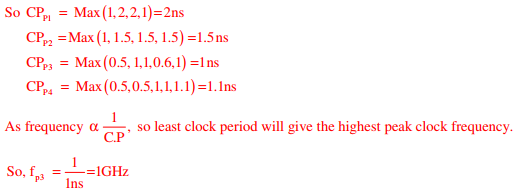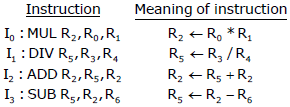# Computer Organization and Architecture - Online Test

Q1. Consider the following processors (ns stands for nanoseconds). Assume that the pipeline registers have zero latency.
P1: Four-stage pipeline with stage latencies 1 ns, 2 ns, 2 ns, 1 ns.
P2: Four-stage pipeline with stage latencies 1 ns, 1.5 ns, 1.5 ns, 1.5 ns.
P3: Five-stage pipeline with stage latencies 0.5 ns, 1 ns, 1 ns, 0.6 ns, 1 ns.
P4: Five-stage pipeline with stage latencies 0.5 ns, 0.5 ns, 1 ns, 1 ns, 1.1 ns.
Which processor has the highest peak clock frequency?

Explaination / Solution:

Clock period (CP) = max stage delay + overheadQ2. Consider the basic COCOMO model where E is the effort applied in person-months, D is the development time in chronological months, KLOC is the estimated number of delivered lines of code (in thousands) and ab ,bb , cb ,db have their usual meanings. The basic COCOMO equations are of the form
Explaination / Solution:
No Explaination.

Q3. A software requirements specification (SRS) document should avoid discussing which one of the following?
Explaination / Solution:

SRS is a description of a software system to be developed, laying out functional & nonfunctional requirements and may include a set of use cases that describe interactions the user will have with the software.

Q4. Consider a processor with byte-addressable memory. Assume that all registers, including Program Counter (PC) and Program Status Word (PSW), are of size 2 bytes. A stack in the main memory is implemented from memory location (0100)16 and it grows upward. The stack pointer (SP) points to the top element of the stack. The current value of SP is (016E)16. The CALL instruction is of two words, the first word is the op-code and the second word is the starting address of the subroutine. (oneword = 2bytes). The CALL instruction is implemented as follows:
 Store the current Vale of PC in the Stack
 Store the value of PSW register in the stack
The content of PC just before the fetch of a CALL instruction is (5FA0)16. After execution of the CALL instruction, the value of the stack pointer is
Explaination / Solution:
No Explaination.

Q5. Host A sends a UDP datagram containing 8880 bytes of user data to host B over an Ethernet LAN. Ethernet frames may carry data up to 1500 bytes (i.e. MTU = 1500 bytes). Size of UDP header is 8 bytes and size of IP heard is 20 bytes.There is no option field in IP header How many total number of IP fragments will be transmitted and what will be the contents of offset field in the last fragment?
Explaination / Solution:Q6. Consider a carry look ahead adder for adding two n-bit integers, built using gates of fan-in at most two. The time to perform addition using this adder is
Explaination / Solution:
No Explaination.

Q7. When two 8-bit numbers A7....A0 and B7.....B0 in 2’s complement representation (with A0 and B0 as the least significant bits ) are added using a ripple-carry adder, the sum bits obtained are S7….S0 and the carry bits are C7….C0. An overflow is said to have occurred if
Explaination / Solution:

Overflow flag indicates an over flow condition for a signed operation. Some points to
remember in a signed operation:
* MSB is always reserved to indicate sign of the number.
* Negative numbers are represented in 2’s – complement.
* An overflow results in invalid operation.
2's complement overflow rules:
* If the sum of two positive numbers yields a negative result, the sum has- overflowed.
* If the sum of two negative number yields a positive result, the sum has overflowed.
* Otherwise, the sum has not overflowed.
Overflow for signed numbers occurs when the carry-in into the MSB (most significant bit) is
not equal to carry-out. Conveniently, an XOR-operation on these two bits can quickly
determine if an overflow condition exists.

Q8. A 5-stage pipelined processor has Instruction Fetch (IF), Instruction Decode (ID), Operand Fetch (OF), Perform Operation (PO) and Write Operand (WO) stages. The IF, ID, OF and WO stages take 1 clock cycle each for any instruction. The PO stage takes 1 clock cycle for ADD and SUB instructions, 3 clock cycles for MUL instruction, and 6 clock cycles for DIV instruction respectively. Operand forwarding is used in the pipeline. What is the number of clock cycles needed to execute the following sequence of instructions?Explaination / Solution:
No Explaination.